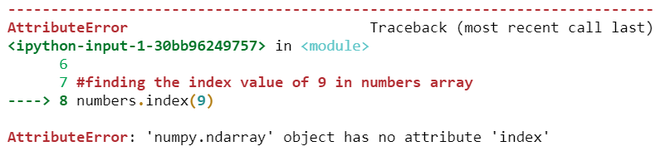# How to Fix: ‘numpy.ndarray’ object has no attribute ‘index’

• Last Updated : 28 Nov, 2021

‘numpy.ndarray’ object has no attribute ‘index’ is an attribute error which indicates that there is no index method or attribute available to use in Numpy array.

This error occurs when we try to find the index of a particular element in a Numpy array using the index method. Below code is an example when that ‘numpy.ndarray’ object has no attribute ‘index’ error can be thrown

Example:

## Python3

 `# import necessary packages``import` `numpy as np`` ` `# Create a Numpy array``numbers ``=` `np.array([``0``, ``1``, ``2``, ``9``, ``8``, ``0``])`` ` `# finding the index value of 9 in ``# numbers array``numbers.index(``9``)`

OutputAs there is no method called index in Numpy it throws an attribute error.

## Solution

To fix this error instead of using index method to find the index of an element use where method which returns an array consists of indexes of a specified element.

Syntax

Numpy.where(arrayName==value_to_find_index)

Example 1:

Specify an element in where method which exist in a Numpy array

## Python3

 `# import necessary packages``import` `numpy as np`` ` `# Create a Numpy array``numbers ``=` `np.array([``0``, ``1``, ``2``, ``9``, ``8``, ``0``])`` ` `# finding the index value of 9 in ``# numbers array``np.where(numbers ``=``=` `9``)`

Output

`(array(, dtype=int64),)`

As Indexes in array starts from 0, Here in the numbers array 0th index consists of value 0, 1st index has value 1, 2nd index has value 2 and 3rd index has value 9 which is specified so it returned an array which contains a value 3.

Example 2:

Specify an element in where method such that the element we specified is occurred more than once in an array.

## Python3

 `# import necessary packages``import` `numpy as np`` ` `# Create a Numpy array``numbers ``=` `np.array([``0``, ``1``, ``2``, ``9``, ``8``, ``0``])`` ` `# finding the index values of 0 in``# numbers array``np.where(numbers ``=``=` `0``)`

Output:

`(array([0, 5], dtype=int64),)`

As element 0 occurred 2 times in numbers array at 0th & 5th index so it return an array consists of 2 index values of element 0.

Example 3:

Pass an element to where method which is actually not in an array.

## Python3

 `# import necessary packages``import` `numpy as np`` ` `# Create a Numpy array``numbers ``=` `np.array([``0``, ``1``, ``2``, ``9``, ``8``, ``0``])`` ` `# finding the index value of a number ``# which is not in numbers array``np.where(numbers ``=``=` `7``)`

Output

`(array([], dtype=int64),)`

If we pass an element to where method which is not in an array then it returns an empty array because there is no specified element at any index of an array. Here 7 is not present in numbers array so it returned an empty array

My Personal Notes arrow_drop_up